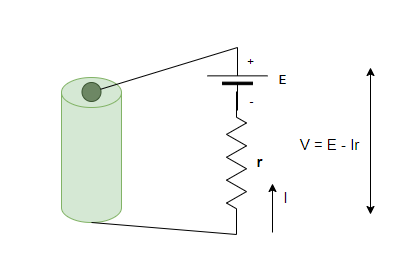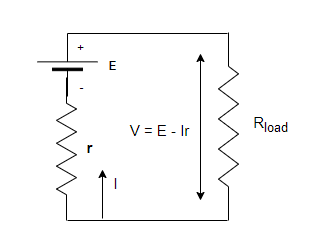Open in App
Not now

# Electromotive Force, Terminal Voltage and Internal Resistance

• Last Updated : 20 Oct, 2021

One can think of many devices which are responsible for creating current in any given circuit. Generators, batteries, wall outlets, etc are necessary to maintain a particular electric current in the circuit. All such devices are essential for maintaining a potential difference across the circuit and are referred to as voltage sources. When a voltage source is connected across a conductor, it creates an electric field which causes the charges to move and this causes current. The values of the current that is generated strictly depends on the characteristic of the material. Any material opposes the flow of electric charge which is called resistance. It is developed due to resistivity which is a property of the material. Voltage sources are also not perfect, they have some internal resistances which reduces their net voltage across terminals. Let’s see the concepts of EMF and internal resistance in detail.

### Electromotive Force, Terminal Voltage and Internal Resistance

When a battery is connected with a bulb, it lights it up. As more and more bulbs are connected to the battery, the intensity of the bulbs decreases. How does it happen? This happens because the output voltage of the battery is decreased. The reason for this can be attributed to two fundamental parts of a battery. A battery has two fundamental parts – electrical energy and internal resistance.

Electromotive Force

All the batteries come in different shapes and sizes. There are many types of generators that are driven by many different sources. All these devices irrespective of what their energy sources are, create potential differences across their terminals and can supply current if resistance is connected to them. It is known that potential difference creates an electric field that causes charges to move and that in turn creates current. Thus, this potential difference is sometimes also referred to as electromotive force (emf).

Contrary to its name, emf is not a force at all. It is a potential difference. If stated in precise terms,

E.M.F is a potential difference which is created when no current is flowing in the system.

Its unit is considered to be all Volts(V). Even though EMF is directly related to the potential difference produced by the voltage source, but it still differs from the actual potential difference that is reflected on the terminals of the battery. The terminal voltage around the battery is usually less than the e.m.f of the battery.

Internal Resistance

It is known that a large emf-based battery has more size than the batteries with less emf. These batteries contain more energy and thus can deliver larger currents. Notice that a 12V battery of a truck can deliver more current than a 12V battery present in a motorcycle. The reason behind this can be attributed to the fact that the battery of the truck has less internal resistance than the battery of a motorcycle.

Internal resistance is the inherent resistance that is present inside a voltage source.The figure above shows two fundamental parts of a voltage source. The emf present inside the battery and the resistance. This emf is denoted by E while the internal resistance is denoted by r, both of them are series. The smaller the internal resistance for the battery, the more current it is able to supply to the circuit. The internal resistance of a battery can behave in complex ways, as the battery depletes the internal resistance of the battery increases. But it may also depend on the magnitude and the direction of the electric current through a voltage source, its temperature, and even the material the battery is made up of.

Terminal Voltage

The voltage output of a battery is measured through its terminals and that is why it is termed as terminal voltage. In the figure given below, a battery and its internal resistance are shown. The battery is connected to another external resistance in series which is denoted by Rload. The net voltage developed across the terminals of the battery is given by the equation written below,

V = emf – IrHere, “I” is the current that is flowing in the circuit, and “r” is the internal resistance.

“I” is considered to be positive if the direction of its flow is from the negative to the positive terminal of the battery. The equation shows that the larger the current, the lower is the terminal voltage of the battery. It can also be concluded that the smaller the internal resistance, the greater is the terminal voltage. When the load resistor is taken into account, the current calculation becomes a little bit different.

The equivalent resistance of the circuit becomes,

The current is given by Ohm’s law,

I =### Sample Problems

Question 1: Find the current that will flow inside the battery of 5 Volts and 0.02 ohms internal resistances in case its terminals are connected with each other.

The current in that case will be given by simple application of ohm’s law.

V = 5V

r = 0.02 ohms.

V = IR

Plugging the values in the equation,

I = V/R

⇒ I = 5/0.02

⇒ I = 250 A

Question 2: Find the current that will flow inside the battery of 10 Volts and 2 ohms internal resistances in case its terminals are connected with each other.

The current in that case will be given by simple application of ohm’s law.

V = 10 V

R = 2 ohms.

V = IR

Plugging the values in the equation,

I = V/R

⇒ I = 10/2

⇒ I = 5 A

Question 3: Find the current that will flow inside the battery of 20 Volts and 5 ohms internal resistances in case its terminals are connected with each other. Find the terminal voltage of the battery.

The current in that case will be given by simple application of ohm’s law.

V = 20 V

R= 5 ohms.

V = IR

Plugging the values in the equation,

I = V/R

⇒ I = 20/5

⇒ I = 4 A

The terminal voltage of the battery is given by,

V = emf – Ir

Given , emf = 20 V, I = 4A and r = 5

V = emf – Ir

⇒ V = 20 – (4)(5)

⇒ V = 0 V

Question 4: Find the current that will flow inside the battery of 20 Volts and 5 ohms internal resistances and 10 ohms load resistance in series. Find the terminal voltage of the battery.

The current in that case will be given by simple application of ohm’s law.

I =emf = 20 V

r = 5

plugging the values in the equation,

I =⇒ I =⇒ I = 1.33 A

The terminal voltage of the battery is given by,

V = emf – Ir

Given , emf = 20 V, I = 4/3 A and r = 5

V = emf – Ir

⇒ V = 20 – (1.33)(5)

⇒ V = 20 – 6.65

⇒ V = 13.35

Question 5: Find the current that will flow inside the battery of 10 Volts and 2 ohms internal resistances and 3 ohms load resistance in series. Find the terminal voltage of the battery.

The current in that case will be given by simple application of ohm’s law.

I =emf = 10 V

r = 2

plugging the values in the equation,

I =⇒ I =⇒ I = 2 A

The terminal voltage of the battery is given by,

V = emf – Ir

Given , emf = 10 V, I =2 A and r = 2

V = emf – Ir

⇒ V = 10 – (2)(2)

⇒ V = 10 – 6

⇒ V = 4V

My Personal Notes arrow_drop_up